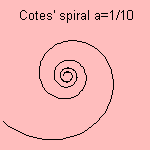# Cotes' spiral

## spiral

last updated: 2003-12-25

The Cotes’ spiral can be defined as the solution for the central orbit problem, where the radial force has the form r = - a |r|-3 r.
The central orbit means that the attractive force between a central mass and a test mass is directed towards that central mass.

The Cotes ’ spiral has three possible solutions, depending on the relation between the value of the constant a and the so-called specific angular momentum 1).

The specific Cotes’ spiral has the following formula:Below I show you some examples of the curve´s nature.
For a=1 you see a double spiral, for the other values for a a single one.notes

1) The square of the momentum h can be defined as: g m s (1-e2), where g is the gravitational constant, m the mass, s the semi-major axis and e the eccentricity.